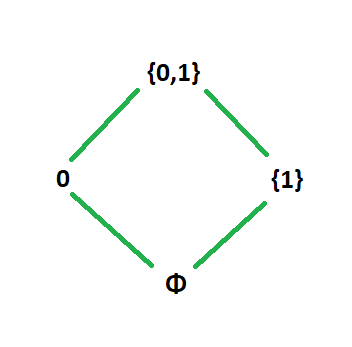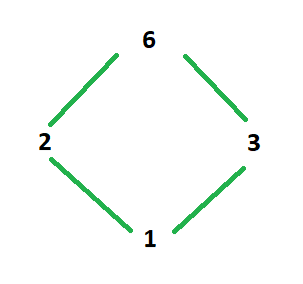# Types of Sets in Discrete Structure or Discrete Mathematics

• Difficulty Level : Medium
• Last Updated : 11 Jan, 2021

In this article, we will discuss Types of Sets in Discrete Structure or Discrete Mathematics. Also, we will cover the examples. Let’s discuss one by one.

1. Partially Ordered Set or POSET :
Partial Ordered Set (POSET) consists of sets with three binary relations as follows.

• Reflexive Relation –One in which every element maps to itself.
• Anti-Symmetric Relation –If (a, b) ∈ R and (b, a) ∈ R, then a=b.
• Transitive Relation –If (a, b) ∈ R and (b, c) ∈ R then (a, c) ∈ R).

Examples –
Let A be a set : A = {1, 2, 3}.

```Question-1 :
R1 = { } .
Is R1 a POSET?
```

R1 is not a POSET because R1 is not reflexive. If any of the three relations is not available then it is not a POSET.

```Question-2 :
R2 = {(1, 1), (2, 2), (3, 3)} .
Is R2 a POSET?
```

This is a POSET because R2 is reflexive, transitive as well as anti-symmetric.

```Question-3 :
R3 = {(1, 1), (2, 2), (3, 3), (1, 2), (2, 1)} .
Is R3 a POSET?```

This is not a POSET because R3 is reflexive but not anti-symmetric. It is actually symmetric. So it cannot be a POSET.

2. Linearly Ordered Set :
It is also known as Chain or Totally Ordered Set. It is basically a POSET in which given any pair (x, y) satisfies either x ≤ y or y ≤ x. Or we can say that if any one of the statements “x<y, y<x, x=y” is correct (This is also called as Law of Trichotomy) then it is a Linearly Ordered Set.

Example –
A set of real numbers with a natural ordering ([R, ≤]) is a Linearly Ordered Set because firstly it is a POSET, and if we take any two real numbers e.g. (r1, r2) ∈ R then at least any one of the following three statements is always true: r1<r2 or r2<r1 or r1=r2. So, it is a Totally or Linearly Ordered Set.

3. Isomorphic Ordered Sets :
Let (A, ≤) and (B, ≤) be two partially ordered sets then they are said to be isomorphic if their “structures” are entirely similar.

Example –
Let two POSETS, A = P({0, 1}) ordered by ≤ and  B = {1, 2, 3, 6} ordered by division relation are Isomorphic Ordered Sets.

Explanation :
Hasse Diagram of POSET A –

`A = { Φ, {0}, {1}, {0, 1} } with subset relation.`Hasse Diagram of POSET B –If we try to define a map.

```f( Φ ) = 1, f( {0} ) = 2,
f( {1} ) = 3 and f( {0, 1} ) = 6.
So both the sets are isomorphic.
Hence, they are Isomorphic Ordered Set.```
4. Well Ordered Set :
A partially ordered set is called a Well Ordered set if every non-empty subset has a least element.

Example –
A set of natural number and less than operation ([N, ≤]) then it is a Well ordered Set because firstly it is a POSET and if we take any two natural numbers e.g. n1 and n2 where n1≤n2. Here, n1 is the least element. So, it is a Well Ordered Set.

Note –

• Any Well ordered set is totally ordered.
• Every subset of a Well ordered set is Well-ordered with the same ordering.
My Personal Notes arrow_drop_up
Recommended Articles
Page :